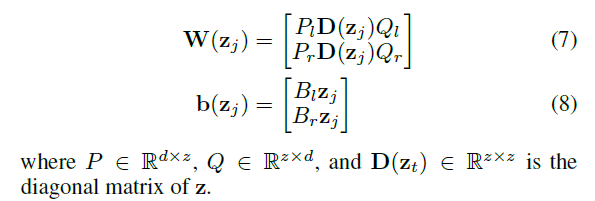# How to compute the diagonal matrix in batch

Hi all,

We have a question that how can we compute PD(z)Q in batch ? z.size() = (batch_size, dim)Thanks.

1 Like

Use torch.diag_embed.

``````torch.diag_embed(input, offset=0, dim1=-2, dim2=-1) → Tensor
``````

Creates a tensor whose diagonals of certain 2D planes (specified by `dim1` and `dim2` ) are filled by `input` . To facilitate creating batched diagonal matrices, the 2D planes formed by the last two dimensions of the returned tensor are chosen by default.

6 Likes